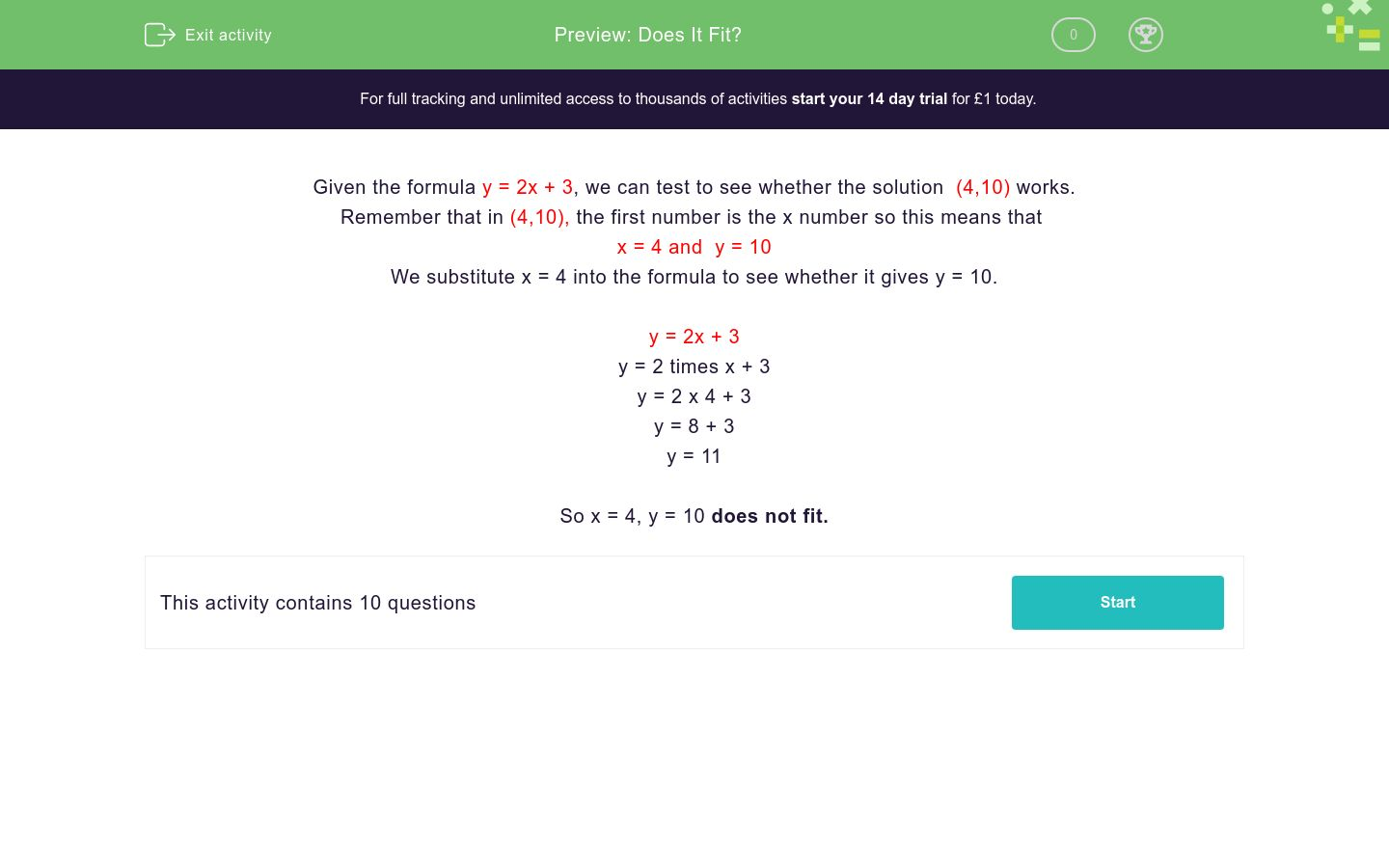# Does It Fit?

In this worksheet, students state whether or not the given solution fits the formula.Key stage:  KS 3

Curriculum topic:   Algebra

Curriculum subtopic:   Use Standard Mathematical Formulae

Difficulty level:#### Worksheet Overview

Given the formula y = 2x + 3, we can test to see whether the solution  (4,10) works.

Remember that in (4,10), the first number is the x number so this means that

x = 4 and  y = 10

We substitute x = 4 into the formula to see whether it gives y = 10.

y = 2x + 3

y = 2 times x + 3

y = 2 x 4 + 3

y = 8 + 3

y = 11

So x = 4, y = 10 does not fit.

### What is EdPlace?

We're your National Curriculum aligned online education content provider helping each child succeed in English, maths and science from year 1 to GCSE. With an EdPlace account you’ll be able to track and measure progress, helping each child achieve their best. We build confidence and attainment by personalising each child’s learning at a level that suits them.

Get started